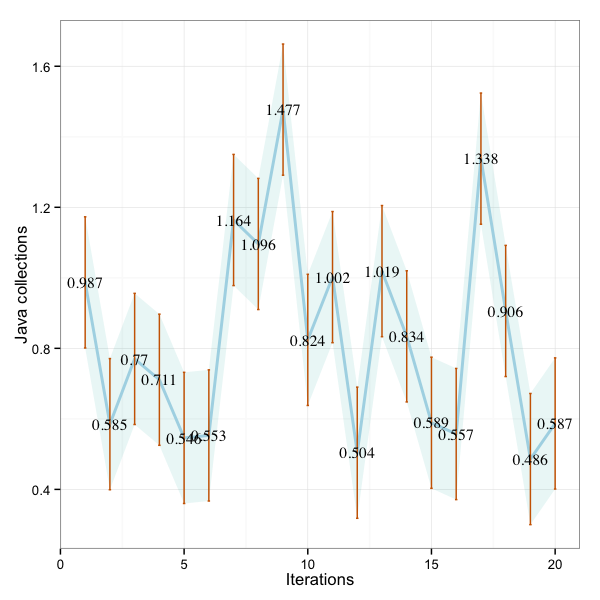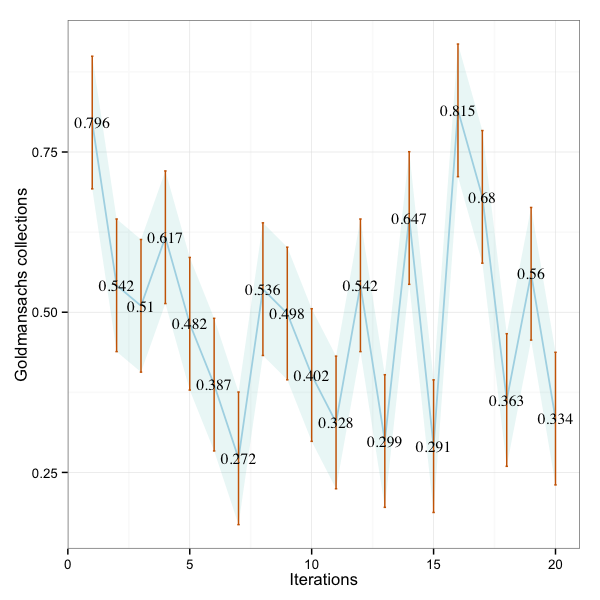R is used to parse the JMH benchmark data and plot these graphs.

## Types of Error bars used to plot the diagram

 Error bars Type Description Standard error (SEM) Inferential A measure of how variable the mean will be, if you repeat the whole study many times. Confidence interval (CI) Inferential A range of values one can be 99% confident contains the true mean.

### Java Collections

R summary

 Min. 1st Qu. Median Mean 3rd Qu. Max. 0.2720 0.3558 0.5040 0.4950 0.5742 0.8150

#### R graph showing error bars and 99% CI as a band### GS Collections

R summary

 Min. 1st Qu. Median Mean 3rd Qu. Max. 0.4860 0.5780 0.7970 0.4950 1.0060 1.4770

#### R graph showing error bars and 99% CI as a band